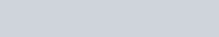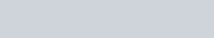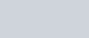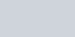You should be familiar with the standard integrals

••In these videos I show you how we can reduce integrals to this form.

Standard Integrals 1/(a^2+x^2) and 1/root(a^2 - x^2) : ExamSolutions Maths Revision - youtube Video

Completing the square and substitution types

I now show you how we can complete the square and use substitution to convert some integrals in to the above forms

Integrals which require completing the square : ExamSolutions Maths Revision - youtube Video

A harder one to try using completing the square leading to tan-1

Integration using completing the square : arctan type (2) : ExamSolutions Maths Revision - youtube Video

A harder one to try using completing the square leading to sin-1

Integration using completing the square : arcsin type (2) : ExamSolutions Maths Revision - youtube Video

Using limits (definite integration)

Care must be taken when it comes to working with limits when using these integrals

Using limits in the integralHow to integrate 1/root(a^2-x^2) with limits : ExamSolutions Maths Revision - youtube Video

Using limits in the integralHow to integrate 1/root(a^2 - x^2) with limits : ExamSolutions Maths Revision - youtube Video